# push()

## A.push()

A.push(chi ,…)

 A 序列 chi 管道

 A 1 =demo.query("select * from SALES") 2 =channel() 创建管道 3 =channel() 创建管道 4 =A2.groups(SELLERID:SellerId;sum(AMOUNT):Amount) A2管道附加结果集函数ch.groups()计算 5 =A3.select(ORDERID<10) A3给管道附加ch.select()计算动作 6 =A3.fetch() A3管道附加结果集函数ch..fetch()函数，保留管道当前数据 7 =A1.push(A2,A3) 将序列A1中的数据推送到管道 8 =A2.result()获取管道计算结果 9 =A3.result()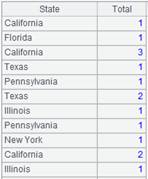获取管道计算结果

## ch.push()

ch.push(chi ,…)

 ch 管道 chi 管道

 A 1 =demo.cursor("select * from SALES") 创建游标 2 =channel() 创建管道 3 =channel() 创建管道 4 =A2.select(ORDERID>100) 给管道附加ch.select()计算动作 5 =A1.push(A2) 将游标A1中的数据推送到管道A2 6 =A2.push(A3) 将管道A2中的数据推送到管道A3 7 =A2.fetch() 管道A2附加结果集函数ch.fetch()，保留管道A2当前数据 8 =A3.groups(SELLERID:SellerId;sum(AMOUNT):Total) 管道A3附加ch.groups()计算动作 9 =A1.groups(SELLERID:SellerId;sum(AMOUNT):Total)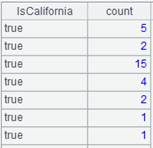A1执行计算，游标中的数据才真正被推送到管道。 10 =A2.result()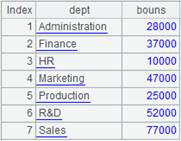获取管道A2计算结果 11 =A3.result()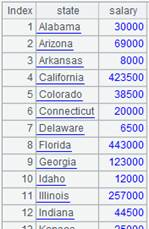获取管道A3计算结果

## cs.push()

cs.push(chi ,…)

 cs 游标 chi 管道

 A 1 =demo.cursor("select * from SALES") 创建游标 2 =channel() 创建管道 3 =channel() 创建管道 4 =A2.groups(SELLERID:SellerId;sum(AMOUNT):Total) 管道执行ch.groups()计算 5 =A3.select(ORDERID<10) 给管道附加ch.select()计算动作 6 =A3.fetch() 保留管道当前数据 7 =A1.push(A2,A3) 将游标A1中的数据推送到管道，此时数据不会立即被推送到管道。 8 =A1.groups(SELLERID:SellerId;sum(AMOUNT):Total)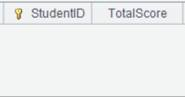A1执行取数计算，游标中的数据才真正被推送到管道。 9 =A2.result()获取管道计算结果 10 =A3.result()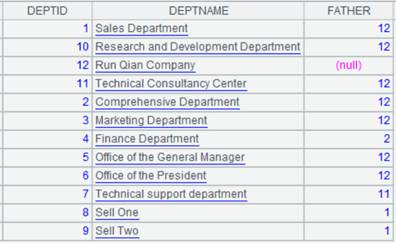获取管道计算结果 A 1 =demo.cursor("select * from SALES") 创建游标 2 =channel() 创建管道 3 =A2.select(ORDERID>100) 给管道附加ch.select()计算动作 4 =A2.fetch() 保留管道当前数据 5 =A1.push(A2) 将游标A1中的数据推送到管道，此时数据不会立即被推送到管道。 6 =A1.fetch()A1执行fetch取数，此时程序才会执行管道中的计算，游标中的数据才真正被推送到管道。 7 =A2.result()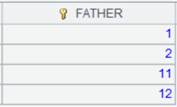获取管道计算结果